# JP4038484B2 - Synchronous phasor measuring device - Google Patents

## Info

Publication number
JP4038484B2
JP4038484B2 JP2004005917A JP2004005917A JP4038484B2 JP 4038484 B2 JP4038484 B2 JP 4038484B2 JP 2004005917 A JP2004005917 A JP 2004005917A JP 2004005917 A JP2004005917 A JP 2004005917A JP 4038484 B2 JP4038484 B2 JP 4038484B2
Authority
JP
Japan
Prior art keywords
timing
voltage
value
phase angle
estimated
Prior art date
Legal status (The legal status is an assumption and is not a legal conclusion. Google has not performed a legal analysis and makes no representation as to the accuracy of the status listed.)
Active
Application number
JP2004005917A
Other languages
Japanese (ja)
Other versions
JP2005204367A (en
Inventor

Original Assignee

Priority date (The priority date is an assumption and is not a legal conclusion. Google has not performed a legal analysis and makes no representation as to the accuracy of the date listed.)
Filing date
Publication date
Application filed by 三菱電機株式会社 filed Critical 三菱電機株式会社
Priority to JP2004005917A priority Critical patent/JP4038484B2/en
Publication of JP2005204367A publication Critical patent/JP2005204367A/en
Application granted granted Critical
Publication of JP4038484B2 publication Critical patent/JP4038484B2/en
Application status is Active legal-status Critical
Anticipated expiration legal-status Critical

## Images

•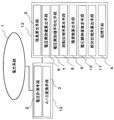•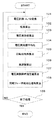••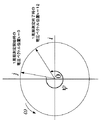•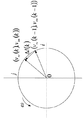•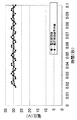•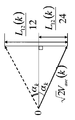••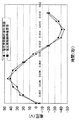•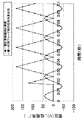•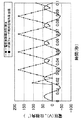•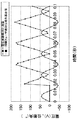•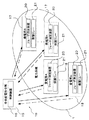•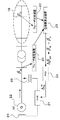•## Classifications

• GPHYSICS
• G01MEASURING; TESTING
• G01RMEASURING ELECTRIC VARIABLES; MEASURING MAGNETIC VARIABLES
• G01R21/00Arrangements for measuring electric power or power factor
• G01R21/133Arrangements for measuring electric power or power factor by using digital technique

## Description

The present invention relates to a synchronized phasor measuring device that measures a synchronized phasor of a power system.

The synchronous phasor measuring device that calculates the amount of electricity from the power system with high accuracy and high speed uses the voltage phasor V using the instantaneous voltage value V k (k = 1 to N) measured at N sampling timings per cycle. Is obtained based on the equation (1). In Equation (1), θ is a sampling angle. Then, the absolute phase angle theta v synchronous phasor from equation (2) (e.g., see Non-Patent Document 1.).

Sekiguchi, 5 others, "Examination of network-type protection control system", IEEJ Transactions B, IEEJ, September 2003, Vol. 123, no. 9, p. 1030-1039

However, the conventional synchronous phasor measurement apparatus, the absolute phase angle theta v is calculated using the tangent function from the real and imaginary parts of the voltage phasors, zero absolute phase angle theta v is from 180 degrees minus, from further zero Change to plus 180 degrees. Then, further absolute phase angle theta v since changes discontinuously from the plus 180 degrees to minus 180 degrees, there is a problem of poor numerical stability with continuity is lost.
Moreover, although the instantaneous voltage value during one cycle is averaged to obtain the effective voltage value, if the frequency to be measured is different from the reference frequency, the effective voltage value and absolute phase angle of the measurement object cannot be measured correctly. . In particular, since the frequency fluctuates greatly when an accident occurs in the power system, there is a problem that the measurement accuracy is poor and the synchronized phasor cannot be used as it is for system protection control that is urgently needed.
There is also a problem that voltage waveform noise due to superposition of harmonic components or instantaneous voltage drop affects the measurement result of the absolute phase angle.

An object of the present invention is to provide a synchronized phasor measuring apparatus capable of measuring the absolute phase angle of a synchronized phasor excellent in continuous numerical stability with high accuracy and high speed in a power system with a lot of noise.

The synchronous phasor measuring device according to the present invention includes a voltage measuring means for measuring the voltage of the power system at a period of 1 / 4N (N is a positive integer) of one period of the reference wave, and at each timing at which the voltage is measured. The voltage effective value calculation means for obtaining the voltage effective value at the own timing from the voltage measured at the past 4N timings including the own timing, and the measured voltage at the own timing at each timing. The real part on the complex plane is obtained, and a voltage rotation vector directed to the point where the measured voltage at the timing delayed by 90 degrees as the electrical angle relative to its own timing is defined as the imaginary part on the complex plane is obtained. At the timing immediately before the leading end of the voltage rotation vector determined in FIG. A chord length calculation means for calculating the chord length of the chord connecting the tip of the pressure rotation vector, and the chord lengths calculated at the past 4N timings including its own timing at each timing are added, Rotation phase for calculating a phase angle between the voltage rotation vector at its own timing and the voltage rotation vector at a timing one cycle before the reference wave from its own timing based on the added value of the length and the average voltage effective value An angle calculating means; a frequency calculating means for calculating the frequency of the power system from the phase angle calculated at each timing; and an angular velocity of a voltage rotation vector from the frequency calculated at the timing at each timing. Each of the measured voltages at the past 4N timings including its own timing. Expanding according to the expression (24), a coefficient estimated value at its own timing is obtained from the coefficients of the past 4N timings including its own timing using the least square method, and the following expression (25) is obtained from the coefficient estimated value. The voltage instantaneous value estimating means for estimating the actual voltage instantaneous number estimated value at its own timing according to the following equation , and the absolute phase angle calculating means for calculating the absolute phase angle of the synchronized phasor at each timing according to the following equation (3).

However, vre is each voltage measured at the past 4N timings including its own timing, ω is the angular velocity obtained at its own timing, P1 and P2 are coefficients at its own timing, and vre_est is an estimation at its own timing The estimated voltage real number instantaneous value, β is the absolute phase angle, vre_est is the estimated voltage real number instantaneous estimated value at its own timing, and V is the effective voltage value calculated at its own timing.

The effect of the synchronized phasor measuring device according to the present invention is that the absolute phase angle of the synchronized phasor is obtained using a cosine function, so that the absolute phase angle increases from zero degree to plus 180 degrees and then reverses to zero degree. Decrease. For this reason, the absolute phase angle is always a positive value and continuously changes at zero degrees or plus 180 degrees, so there is no discontinuous change as in the past and the amount of change is small. Excellent in continuity and continuity.

Embodiment 1 FIG.
FIG. 1 is a block diagram of a synchronous phasor measuring apparatus according to Embodiment 1 of the present invention. FIG. 2 is a flowchart of synchronous phasor measurement in FIG.

The synchronous phasor measuring device includes a voltage measuring unit 2 that measures a voltage real number instantaneous value of the power system 1 at a predetermined timing, and an A / D conversion unit that converts the measured voltage real number instantaneous value into a digital voltage real number instantaneous value. 3, a storage means 4 for storing a digital voltage real number instantaneous value, a chord length calculation means 5 for calculating the length of a chord formed between the tips of adjacent voltage rotation vectors at every predetermined timing, and a voltage rotation vector Voltage effective value calculating means 6 for calculating the voltage effective value from the above, voltage effective value averaging means 7 for calculating the voltage effective value average value by averaging the calculated voltage effective value over a predetermined period, and the calculated string A rotation phase angle calculation means 8 for calculating the rotation phase angle by integrating the length of the power supply over a predetermined period, a frequency calculation means 9 for calculating the frequency of the power system from the calculated rotation phase angle, and a digital voltage Real number A voltage instantaneous value estimation means 10 for calculating a voltage real instantaneous estimate using the least square method from the time value and the absolute phase angle calculating means 11 for calculating the absolute phase angle of the synchronous phasor.

The voltage measuring means 2 and the A / D conversion means 3 are composed of a voltmeter 12 having a digital voltage output terminal. Furthermore, the storage means 4, the chord length calculation means 5, the voltage effective value calculation means 6, the voltage effective value averaging means 7, the rotation phase angle calculation means 8, the frequency calculation means 9, the voltage instantaneous value estimation means 10 and the absolute phase angle calculation. The means 11 is constituted by a computer 13. The computer 13 has a CPU, a RAM, a ROM, and an interface circuit.
Each synchronized phasor measuring device synchronizes clocks using GPS.

In the following description, the frequency f 0 (Hz) of the reference wave will be described by taking 50 Hz and 60 Hz as an example, but is not limited to this frequency. One period (second) of the reference wave is the reciprocal 1 / f 0 of the frequency f 0 .

Furthermore, the time point (hereinafter referred to as “timing”) at which the voltage real number instantaneous value is measured is determined by determining the sampling period. The sampling period may be a value that can equally divide one period of the reference wave into 4N (N is a positive integer). As will be described later, since the voltage real number instantaneous value at the time when it is delayed by 90 degrees is used as the coordinates of the tip of the voltage rotation vector, it is necessary that the time when it is delayed by 90 degrees is one of the timings. One period of the reference wave is 2π (radian) expressed in electrical angle. For example, the electrical angle π / 6, π / 12, π / 24, π / 48, etc. of the reference wave is set in advance as the sampling period.

Next, the procedure of the synchronized phasor measurement of the power system will be described with reference to FIG. In this description, the frequency f 0 of the reference wave is 50 Hz, and π / 6 that can divide one period of the reference wave into 12 equal parts (N = 3) is the sampling period. The step size Δt (seconds) is 0.00166667 seconds. k represents the order of voltage measurement timing, and is 1 to 12 in one cycle of the reference wave. The reference wave electrical angle is rotated by 30 degrees between the voltage measurement timings k = 1 and k = 2.

In step 101, the voltage measuring means 2 measures the voltage of the power system to be measured at each voltage measurement timing. Arbitrary timing of voltage measurement timing is represented by k. The timing one time before this timing can be represented by (k−1), and the next timing can be represented by (k + 1). Next, the A / D conversion means 3 performs A / D conversion of the measured voltage, which is an analog signal, into a digital voltage signal. This value is stored in the storage means 4. As shown in FIG. 3, when the voltage instantaneous value v (k) of the power system is expressed by a voltage rotation vector that rotates counterclockwise around the origin 0 on the complex plane, the measured voltage is expressed by the equation (4). ) Is a voltage real number instantaneous value v re (k). Here, V is an effective voltage value (volt), ω is an angular velocity (radian / second) of the voltage rotation vector, and φ 0 is a voltage initial rotation phase angle (radian). ω has a relationship of ω = 2πf with the frequency f of the power system to be measured.

In step 102, the chord length calculation means 5 uses the voltage measured at each timing as the real part on the complex plane at each timing of the voltage measurement timing, and is delayed by 90 degrees as an electrical angle with respect to each timing. A voltage rotation vector at each timing directed to a point where the voltage measured at the relevant timing is an imaginary part on the complex plane is obtained. Next, at each timing of the voltage measurement timing, the length of the string connecting the tip of the voltage rotation vector obtained at each timing and the tip of the voltage rotation vector obtained at the timing immediately before each timing is determined. calculate. As shown in FIG. 4, the voltage rotation vector at a predetermined timing of the power system to be measured has a phase angle in the counterclockwise direction in the complex plane during one period of the reference wave, that is, between k = 0 and k = 12. Rotate by Ψ (radians). Next, as shown in Expression (4), the voltage instantaneous value v (k) is composed of a voltage real number instantaneous value v re (k) and a voltage imaginary number instantaneous value v im (k). This voltage imaginary instantaneous value v im (k) is equal to the voltage real number instantaneous value v re (k−3) as shown in Expression (5). When the sampling period is π / 6, the voltage is measured at the timing (k-3). For example, when π / 12 is used, the voltage is measured at the timing (k-6). In this way, the coordinates of the tip of the voltage rotation vector can be represented by the voltage real number instantaneous value v re (k) and the voltage imaginary number instantaneous value v im (k). The voltage imaginary instantaneous value v im (k) is obtained by reading out the corresponding voltage real number instantaneous value v re (k−3) from the storage unit 4. In this way, since the coordinates of the respective voltage rotation vectors at timings k and (k−1) can be obtained, the length Δl (k) of the string connecting the tips of the voltage rotation vectors shown in FIG. (6).

In step 103, the voltage effective value calculation means 6 calculates the voltage of one cycle of the reference wave from the voltage measured at the previous 12 timings including its own timing at each timing of the voltage measurement timing. A voltage effective value V (k) is calculated from the equation (7) using the voltage real number instantaneous value vre .

In step 104, the voltage effective value averaging means 7 at each timing of the voltage measurement timing, from the voltage effective value calculated at its own timing and the voltage effective value calculated at 11 timings prior to its own timing. An average value V ave (k) of the voltage effective value V during one period of the reference wave is calculated according to the equation (8). The average value V ave (k) of the voltage effective value at its own timing k is the voltage effective value average value obtained at each of its own timing k from the timing (k-11). As shown in FIG. 6, when the frequency of the power system to be measured is different from the frequency of the reference wave, the voltage effective value oscillates around the actual voltage effective value. In order to remove the influence of this vibration, an averaging process is performed.

The longer the averaging processing time is, the more accurate the voltage effective value is. However, in order to shorten the measurement time, the voltage effective value obtained in one sampling cycle, in this case, 12 times of sampling is used. To perform the averaging process. When the voltage effective values are averaged in this way, the influence of the difference between the frequency of the power system to be measured and the frequency of the reference wave can be excluded as shown in FIG.

In step 105, the rotation phase angle calculation means 8 uses the formula (9) to calculate the chord length calculated at the past 12 timings from its own timing, including its own timing, at each timing of the voltage measurement timing. Find the total chord length L 12 (k). Next, as shown in FIG. 7, the rotation phase angle calculation means 8 rotates between its own timing k and the timing (k-1) immediately before its own timing, as shown in FIG. the phase angle 2.alpha k is calculated according to the equation (10). Next, at each timing of the voltage measurement timing, the phase between the voltage rotation vector at its own timing k from the rotation phase angle 2α k and the voltage rotation vector at the timing one cycle before the reference wave from its own timing k. The angle Ψ (k) is calculated by the equation (11). The phase angle Ψ (k) is an electrical angle obtained by rotating the voltage rotation vector in one cycle of the reference wave.

In step 106, the frequency calculation means 9 determines the frequency f ((f) of the power system to be measured from the phase angle 2π of one cycle of the reference wave and the phase angle Ψ (k) of the measurement object at each timing of the voltage measurement timing. k) is calculated using equation (12). f 0 is the frequency of the reference wave.

In step 107, the voltage instantaneous value estimating means 10 measures the voltage actual number instantaneous estimated value at its own timing at the timing of the past 12 times from its own timing including its own timing at each timing of the voltage measurement timing. The obtained voltage real number instantaneous value v re is obtained using the least square method. The real voltage instantaneous value v re (t) is expanded by the sine and cosine of the product of the angular velocity and time as shown in the equation (13), and the coefficients of the sine and cosine can be rewritten as P 1 and P 2. it can. Then, the coefficient estimated values P 1est and P 2est of the coefficients P 1 and P 2 can be obtained, and the voltage real number instantaneous estimated value can be obtained therefrom . The least square method is used for estimating the coefficient estimation values P 1est and P 2est . The angular velocity ω is obtained from the frequency f (k).
Determining the voltage measured at the previous 12 timings from its own timing k including its own timing k according to the equation (13) can be expressed as the determinant (14). Furthermore, the voltage real number instantaneous value matrix [v] and the trigonometric function matrix [A] can be expressed by determinants (15) and (16), respectively. The sampling timing time matrix can be expressed by determinant (17). Δt is a calculation step, and is 0.0016667 s when the reference wave is 50 Hz and divides one cycle into twelve, and 0.00168889 s when the reference wave is 60 Hz and one cycle is divided into twelve. The arbitrary coefficient matrix [P] can be expressed by the determinant (18).
When each matrix is expressed in this way, determinant (14) can be expressed as determinant (19). The arbitrary coefficient matrix [P] can be obtained by the determinant (20) using the least square method. Here, the transposed matrix [A] T is the determinant (21). The estimated values of the arbitrary coefficient matrix [P] are the coefficient estimated values P 1est and P 2est .

Since the arbitrary constant matrix [P] can be obtained in this way, the voltage real number instantaneous estimated value v re_est (k) can be obtained as Expression (22). FIG. 9 shows the measured value of the voltage real number instantaneous value and the voltage real number instantaneous value estimated using the least square method. As can be seen from FIG. 9, even if the voltage real number instantaneous value fluctuates due to voltage waveform noise or the like, since the estimation is performed using the least square method, the influence of the voltage waveform noise reaches this voltage real number instantaneous estimated value. Is gone.
Next, in step 108, the absolute phase angle calculation means 11 at each timing of the voltage measurement timing, the voltage effective value average value obtained by the equation (8) and the voltage real number instantaneous estimated value obtained by the equation (22). Then, the absolute phase angle β (k) is obtained by the equation (23). In addition, you may obtain | require an absolute phase angle using the voltage measured at each timing instead of a voltage real number instantaneous estimated value. Moreover, you may obtain | require an absolute phase angle using the voltage effective value calculated | required by Formula (7) instead of the voltage effective value average value.

In step 109, it is determined whether or not the measurement of the absolute phase angle of the synchronized phasor of the power system is to be terminated.

Next, the absolute phase angle of the synchronized phasor was calculated when the frequency of the power system was 50 Hz, 55 Hz, and 45 Hz when the reference wave was 50 Hz and one cycle was divided into 12 equal parts. FIG. 10 shows the results at 50 Hz, FIG. 11 at 55 Hz, and FIG. 12 at 45 Hz. As can be seen from these figures, the absolute phase angle of the synchronized phasor can be measured with high accuracy even when the frequency of the power system fluctuates. Since the actual frequency of the electric power system has a maximum fluctuation of 1 to 2 HZ around the reference frequency, the synchronous phasor measuring device of the present invention can measure a highly accurate and stable synchronous phasor.

The calculation step width Δt is set to the reference wave rotation electrical angle of 30 degrees. However, when actual measurement is performed, the smaller the calculation step width Δt, the higher the measurement accuracy.

In such a synchronized phasor measuring device of the power system, the absolute phase angle of the synchronized phasor is obtained using a cosine function, so that the absolute phase angle increases from zero degree to plus 180 degrees, and then reverses to zero degree. Decrease. For this reason, the absolute phase angle is always a positive value and continuously changes at zero degrees or plus 180 degrees, so there is no discontinuous change as in the past and the amount of change is small. Excellent in continuity and continuity.

In addition, a voltage rotation vector is obtained by using a voltage obtained by measuring one period of the reference wave with a period of 1 / N. On the other hand, a frequency is obtained from a comparison between the rotation phase angle of the voltage rotation vector and the rotation phase angle of the reference wave. On the other hand, since the voltage real number instantaneous estimated value is estimated from the measured voltage real number instantaneous value, the synchronous phasor can be measured in a short time of one cycle of the power system.

Further, since the moving average of the voltage effective value is obtained, the error of the voltage effective value is reduced, and the measurement error of the absolute phase angle of the synchronous phasor obtained using this voltage effective value is also reduced. When an accident occurs in the actual power system, the frequency changes greatly, but the effect can be minimized.

In addition, since the voltage real number instantaneous estimated value is estimated from the measured voltage using the least square method, when the load fluctuates or the voltage changes sharply, these effects are suppressed and the absolute phase angle The calculation error can be reduced.

In the present invention, the voltage is expressed by a voltage vector that rotates on a complex plane. However, in order to express a steady state of voltage in the conventional AC theory, it may be expressed by a static vector (phasor). Is different.

Embodiment 2. FIG.
FIG. 13 is a block diagram of a power system protection control system provided with a synchronized phasor measuring device according to Embodiment 2 of the present invention at each power plant and substation.
The power system protection control system is provided in the central power supply station 15, and is provided in the control device 16 that monitors and controls the entire power system 1, and the power plant 17 and the substation 18 that are arranged in each place of the power system 1. A synchronized phasor measuring device 20 that measures the synchronized phasor of the system and transmits the value to the control device 16 via the network 19, and a protection relay 21 that is provided in the power plant 17 and the substation 18 and protects and controls the power system 1. And have. Note that the synchronized phasor measuring device 20 is the same as that of the first embodiment, and a description thereof will be omitted. Each synchronized phasor measuring device 20 is synchronized using GPS.

Measurement information including the absolute phase angle and frequency of the synchronized phasor measured at each power plant 17 and substation 18 is sent from the substation 18 and power plant 17 to the control device 16 via the network 19 at a constant cycle, for example, one cycle. In the case of 50 Hz, transfer is performed within 20 ms, and the control device 16 comprehensively determines the difference in absolute phase angle and the variation in frequency, and generates a power generation limit command such as a generator trip to each power plant 17. 18 sends a load limit or system separation command. In accordance with these instructions, the protection relay 21 is activated to protect the power system 1.

Such a power system protection control system enables stable operation of the power system and can prevent a large-scale power outage.

Embodiment 3 FIG.
FIG. 14 is a block diagram of a power system long period fluctuation control device including a synchronous phasor measurement device according to Embodiment 3 of the present invention.
The power system length periodic oscillation control device includes a synchronized phasor measuring device 20 that measures a synchronized phasor at the end of the generator 22, and a synchronized phasor measurement that measures a synchronized phasor of a substation 18 disposed far from the generator 22. A phase difference calculating device 23 for calculating a phase difference from an absolute phase angle from each synchronous phasor measuring device 20, a stabilization control device (Power System Stabilizer) 24 for attenuating long-period fluctuations in the system, and a transformer And an automatic voltage regulator 27 for controlling the excitation circuit 26 of the generator 22 using the terminal voltage and phase angle of the generator 22 measured at 25.

The operation of this power system long period fluctuation control device will be described. The synchronous phasor measuring device 20 provided at the own end of the generator 22 and at the substation 18 is the same as that of the first embodiment, and the description thereof is omitted.
The phasor measuring device 20 measures the absolute phase angle β M at the end of the generator 22 and the absolute phase angle β N at the substation 18 arranged far away. Since these absolute phase angles β M and β N are synchronized by GPS, the phase difference calculation device 23 calculates the phase difference Δβ MN at the same time. This phase difference Δβ MN is input to the stabilization controller 24, and a control signal Δζ for the automatic voltage regulator 27 is calculated based on the phase difference Δβ MN . In the automatic voltage regulator 27, the voltage of the transmission line is detected by the transformer 25, and the excitation current of the excitation circuit 26 is controlled based on the voltage and the control signal Δζ. In this way, the generated power of the generator 22 is controlled when a long-period fluctuation occurs.

Such a power system long-period oscillation control device has a longer-period oscillation than when a long-period oscillation generated in the power system is used for the control by the stabilization control device 24 using a conventional active power variation or frequency variation. Can be reduced.

Embodiment 4 FIG.
FIG. 15 is a block diagram of a power system step-out detection relay protection device including a synchronous phasor measurement device according to Embodiment 4 of the present invention.

The power system step-out detection relay protection device includes a synchronous phasor measurement device (PMU) 20, a protection relay (Ry) 21, and a circuit breaker (CB) at substations A18 and B18 disposed at both ends of the transmission line 30, respectively. ) 31 is provided. A generator GA22 and a generator GB22 are connected to the substation A18 and the substation B18, respectively.
Next, a procedure for determining that the power system is out of step and tripping the circuit breaker 31 will be described. The protection relay 21 transmits the absolute phase difference β A and β B measured by the synchronous phasor measurement device 20 to the protection relay 21 at the other end via the PCM communication channel 32. The protection relay 21 obtains a phase difference Δβ AB = β A −β B from the other end, and determines that the step-out occurs when the phase difference Δβ AB exceeds a predetermined threshold value β TH . Then, the protection relay 21 trips the circuit breaker 31.

Such a power system step-out detection relay protection device can measure the synchronized phasor absolute phase angle with high accuracy, high speed, and stability, so it adopts a new operating principle of detecting step out from phase difference. Can be detected.

It is a block diagram of the synchronous phasor measuring device concerning Embodiment 1 of this invention. It is a flowchart of the synchronous phasor measurement procedure of FIG. It is the voltage rotation vector figure expressed on the complex number plane. It is a figure which shows a mode that it rotated during 1 period of the reference | standard wave of FIG. It is a figure explaining calculation of the chord length between two points of the tip of a voltage rotation vector. It is a measurement result of the voltage effective value of the power system of the frequency different from the frequency of the reference wave measured in FIG. It is a figure explaining the calculation of the phase angle which faced the chord which the front-end | tip of a voltage rotation vector forms. It is a right triangle which shows an absolute phase angle with a voltage effective value and a voltage real number instantaneous value estimated value. The result which calculated | required the voltage real number instantaneous value estimated value from the voltage real number instantaneous value measured value is shown. It is an absolute phase angle measurement result of the electric power system of frequency 50Hz. (Reference wave frequency is 50Hz) It is an absolute phase angle measurement result of a power system with a frequency of 55 Hz. (Reference wave frequency is 50Hz) It is an absolute phase angle measurement result of the electric power system of frequency 45Hz. (Reference wave frequency is 50Hz) It is a block diagram of the electric power system stabilization protection apparatus using the synchronous phasor measuring device of Embodiment 2 of this invention. It is a block diagram of an electric power system length periodic fluctuation control device using the synchronous phasor measuring device of Embodiment 3 of this invention. It is a block diagram of the electric power system step-out detection relay protection apparatus using the synchronous phasor measuring device of Embodiment 4 of this invention.

Explanation of symbols

1 power system, 2 voltage measurement means, 3 A / D conversion means, 4 storage means, 5 chord length calculation means, 6 voltage effective value calculation means, 7 voltage effective value averaging means, 8 rotational phase angle calculation means, 9 frequency Calculation means, 10 voltage instantaneous value estimation means, 11 absolute phase angle calculation means, 12 voltmeter, 13 computer, 15 central power supply command station, 16 control device, 17 power plant, 18 substation, 19 network, 20 synchronous phasor measurement device , 21 Protection relay, 22 Generator, 23 Phase difference calculation device, 24 Stabilization control device (PPS), 25 Transformer, 26 Excitation circuit, 27 Automatic voltage regulator, 30 Power transmission line, 31 Circuit breaker, 32 PCM communication channel .

## Claims (3)

1. Voltage measuring means for measuring the voltage of the power system at a period of 1 / 4N (N is a positive integer) of one period of the reference wave;
At each timing at which the voltage is measured, voltage effective value calculation means for obtaining a voltage effective value at the own timing from the voltage measured at the past 4N timings including the own timing;
At each timing, the voltage measured at its own timing is the real part on the complex plane, and the voltage measured at the timing delayed by 90 degrees as the electrical angle with respect to its own timing is the imaginary part on the complex plane. The chord length of the string connecting the leading end of the voltage rotation vector determined at its own timing and the leading end of the voltage rotation vector at the timing immediately before its own timing Chord length calculating means for calculating
At each timing, the chord length calculated at the past 4N timings including its own timing is added, and the voltage at its own timing is calculated based on the added value of the chord length and the average voltage effective value. A rotation phase angle calculating means for calculating a phase angle between the rotation vector and the voltage rotation vector at a timing one cycle before the reference wave from its own timing;
Frequency calculating means for calculating the frequency of the power system from the calculated phase angle at each timing;
At each timing, the angular velocity of the voltage rotation vector is obtained from the frequency calculated at its own timing, and each voltage measured at the past 4N timings including its own timing is obtained.

(Where vre is each voltage measured at the past 4N timings including its own timing, ω is the angular velocity obtained at its own timing, and P1 and P2 are coefficients at its own timing)
The coefficient estimation value at its own timing is obtained from the coefficients of the past 4N timings including its own timing using the least square method, and the coefficient estimation value is

(Where vre_est is an estimated voltage real number instantaneous estimated value at its own timing, and P1est and P2est are estimated coefficient estimated values at its own timing)
A voltage instantaneous value estimating means for estimating a voltage real number instantaneous estimated value at its own timing according to:

(Where β is the absolute phase angle, vre_est is the estimated voltage real number instantaneous value estimated at its own timing, and V is the calculated voltage effective value at its own timing)
Absolute phase angle calculating means for calculating the absolute phase angle of the synchronized phasor at each timing according to the above,
A synchronized phasor measuring apparatus comprising:
2. Voltage measuring means for measuring the voltage of the power system at a period of 1 / 4N (N is a positive integer) of one period of the reference wave;
At each timing at which the voltage is measured, voltage effective value calculation means for obtaining a voltage effective value at the own timing from the voltage measured at the past 4N timings including the own timing;
At each of the above timings, the voltage effective value having an average value of the voltage effective value calculated at its own timing and the voltage effective value calculated at at least one preceding timing as the voltage effective value average value at its own timing. Value averaging means;
At each timing, the voltage measured at its own timing is the real part on the complex plane, and the voltage measured at the timing delayed by 90 degrees as the electrical angle with respect to its own timing is the imaginary part on the complex plane. The chord length of the string connecting the leading end of the voltage rotation vector determined at its own timing and the leading end of the voltage rotation vector at the timing immediately before its own timing Chord length calculating means for calculating
At each timing, the chord length calculated at the past 4N timings including its own timing is added, and the voltage at its own timing is calculated based on the added value of the chord length and the average voltage effective value. A rotation phase angle calculating means for calculating a phase angle between the rotation vector and the voltage rotation vector at a timing one cycle before the reference wave from its own timing;
Frequency calculating means for calculating the frequency of the power system from the calculated phase angle at each timing;
At each timing, the angular velocity of the voltage rotation vector is obtained from the frequency calculated at its own timing, and each voltage measured at the past 4N timings including its own timing is obtained.

(Where vre is each voltage measured at the past 4N timings including its own timing, ω is the angular velocity obtained at its own timing, and P1 and P2 are coefficients at its own timing)
The coefficient estimation value at its own timing is obtained from the coefficients of the past 4N timings including its own timing using the least square method, and the coefficient estimation value is

(Where vre_est is an estimated voltage real number instantaneous estimated value at its own timing, and P1est and P2est are estimated coefficient estimated values at its own timing)
A voltage instantaneous value estimating means for estimating a voltage real number instantaneous estimated value at its own timing according to:

(Where β is the absolute phase angle, vre_est is the estimated instantaneous voltage value at its own timing, and Vave is the average voltage effective value calculated at its own timing)
Absolute phase angle calculating means for calculating the absolute phase angle of the synchronized phasor at each timing according to the above,
A synchronized phasor measuring apparatus comprising:
3. Power system stabilizing protection device, synchronous phasor measurement device according to claim 1 or 2, characterized in that provided in one of the power system long period fluctuation control device and an electric power system out detection relay protection device .
JP2004005917A 2004-01-13 2004-01-13 Synchronous phasor measuring device Active JP4038484B2 (en)

## Priority Applications (1)

Application Number Priority Date Filing Date Title
JP2004005917A JP4038484B2 (en) 2004-01-13 2004-01-13 Synchronous phasor measuring device

## Applications Claiming Priority (3)

Application Number Priority Date Filing Date Title
JP2004005917A JP4038484B2 (en) 2004-01-13 2004-01-13 Synchronous phasor measuring device
US10/873,177 US7006935B2 (en) 2004-01-13 2004-06-23 Synchronous vector measuring device
FR0407487A FR2865038B1 (en) 2004-01-13 2004-07-06 Device for measuring a synchronous vector

## Publications (2)

Publication Number Publication Date
JP2005204367A JP2005204367A (en) 2005-07-28
JP4038484B2 true JP4038484B2 (en) 2008-01-23

# Family

## Family Applications (1)

Application Number Title Priority Date Filing Date
JP2004005917A Active JP4038484B2 (en) 2004-01-13 2004-01-13 Synchronous phasor measuring device

## Country Status (3)

US (1) US7006935B2 (en)
JP (1) JP4038484B2 (en)
FR (1) FR2865038B1 (en)

## Families Citing this family (26)

* Cited by examiner, † Cited by third party
Publication number Priority date Publication date Assignee Title
JP4648848B2 (en) * 2006-02-06 2011-03-09 三菱電機株式会社 Synchronizing device
JP4657151B2 (en) * 2006-06-01 2011-03-23 三菱電機株式会社 Rotational phase angle measuring device, frequency measuring device using the same, synchronous phasor measuring device, switching pole phase control device, synchronous input device and phase discrimination device
US7710693B2 (en) * 2006-09-22 2010-05-04 Schweitzer Engineering Laboratories, Inc. Apparatus and method for providing protection for a synchronous electrical generator in a power system
CN101479612B (en) * 2007-02-19 2011-09-14 三菱电机株式会社 Frequency measuring instrument
EP2124068A4 (en) * 2007-02-27 2010-08-04 Mitsubishi Electric Corp Ac electric quantity measuring device
JPWO2008126240A1 (en) 2007-03-30 2010-07-22 三菱電機株式会社 Synchronous phasor measuring device and phase angle difference measuring device between buses using the same
JP5214163B2 (en) * 2007-03-30 2013-06-19 北海道旅客鉄道株式会社 Phase difference measurement system and phase difference measurement method
JP4818188B2 (en) * 2007-04-18 2011-11-16 三菱電機株式会社 Frequency change measuring device, frequency change rate measuring device, and power system control protection device
MX2010002165A (en) * 2007-09-28 2010-06-02 Schweitzer Engineering Lab Inc Systems and methods for power swing and out-of- step detection using time stamped data.
JP2009122057A (en) * 2007-11-19 2009-06-04 Canon Inc Measurement apparatus
US7979220B2 (en) * 2008-08-21 2011-07-12 Abb Research Ltd. System and method for monitoring power damping compliance of a power generation unit
JP5188449B2 (en) * 2009-05-18 2013-04-24 三菱電機株式会社 Synchronous phasor measuring device
DE102009031017B4 (en) * 2009-06-29 2018-06-21 Wobben Properties Gmbh Method and device for monitoring a three-phase alternating voltage network and wind energy plant
US8554385B2 (en) * 2009-09-11 2013-10-08 Schweitzer Engineering Laboratories Inc. Systems and methods for monitoring and controlling electrical system stability
US8405940B2 (en) * 2009-10-13 2013-03-26 Schweitzer Engineering Laboratories Inc Systems and methods for generator ground fault protection
JP5501933B2 (en) * 2010-10-25 2014-05-28 三菱電機株式会社 AC electric quantity measuring device and AC electric quantity measuring method
CN102064524B (en) * 2010-11-10 2014-03-12 中电普瑞科技有限公司 Electrical power system desynchronization and rapid disconnection judging method
JP5538203B2 (en) * 2010-12-27 2014-07-02 三菱電機株式会社 Power fluctuation detection device and power fluctuation detection method
CN102520246B (en) * 2011-12-05 2014-03-12 西安交通大学 Constant frequency phasor extraction method
JP6049469B2 (en) * 2013-01-21 2016-12-21 三菱電機株式会社 Electric quantity measuring apparatus and electric quantity measuring method, and power system quality monitoring apparatus, three-phase circuit measuring apparatus, electric power system step-out prediction apparatus, active filter and switching pole phase control apparatus using these apparatuses and methods
FR3001807B1 (en) * 2013-02-07 2016-06-10 Renault Sa Method for determining the average voltage of the cells of a battery, in particular a high voltage battery for an electric vehicle
CN104155521A (en) * 2013-05-15 2014-11-19 国家电网公司 Method and apparatus for determining phase difference
US10288688B2 (en) 2014-07-24 2019-05-14 Schweitzer Engineering Laboratories, Inc. Systems and methods for monitoring and protecting an electric power generator
US9496707B2 (en) 2014-12-22 2016-11-15 Schweitzer Engineering Laboratories, Inc. Generator protection element
US10333291B2 (en) 2017-09-25 2019-06-25 Schweitzer Engineering Laboratories, Inc. Multiple generator ground fault detection
CN108802540A (en) * 2018-07-19 2018-11-13 山东大学 Method for visualizing suitable for power distribution network synchronized phasor real-time state monitoring

## Family Cites Families (8)

* Cited by examiner, † Cited by third party
Publication number Priority date Publication date Assignee Title
US3723718A (en) * 1970-11-09 1973-03-27 Syst De Corp Simulation through rotating coordinate transformation
US5006846A (en) * 1987-11-12 1991-04-09 Granville J Michael Power transmission line monitoring system
US5568154A (en) * 1995-05-22 1996-10-22 State Of Israel-Ministry Of Defense Armament Development Authority-Rafael System and a method for the instantaneous determination of the frequencies and angles of arrival of simultaneously incoming RF signals
US5673196A (en) * 1995-11-30 1997-09-30 General Electric Company Vector electricity meters and associated vector electricity metering methods
EP1211775B1 (en) * 2000-12-01 2004-07-14 ABB Schweiz AG Method and device for assessing the stability of an electric power transmission network
GB2382662B (en) * 2001-11-29 2003-12-10 Univ Cardiff High frequency circuit analyzer
US20030115008A1 (en) * 2001-12-18 2003-06-19 Yutaka Doi Test fixture with adjustable pitch for network measurement
KR20040077722A (en) * 2002-01-15 2004-09-06 아가매트릭스, 인코포레이티드 Method and apparatus for processing electrochemical signals

## Also Published As

Publication number Publication date
FR2865038B1 (en) 2006-03-24
FR2865038A1 (en) 2005-07-15
US20050151532A1 (en) 2005-07-14
US7006935B2 (en) 2006-02-28
JP2005204367A (en) 2005-07-28

## Similar Documents

Publication Publication Date Title
US7096175B2 (en) Stability prediction for an electric power network
US6662124B2 (en) Protective relay with synchronized phasor measurement capability for use in electric power systems
US20100213925A1 (en) Advanced real-time grid monitoring system and method
Chakrabarti et al. Measurements get together
Singh et al. Decentralized dynamic state estimation in power systems using unscented transformation
EP2963759B1 (en) Power conversion device for connection to grid
Benmouyal et al. Zero-setting power-swing blocking protection
US6996483B2 (en) System and method for locating a disturbance in a power system based upon disturbance power and energy
RU2464581C2 (en) Measurement of full resistance of power transmission line
US7930117B2 (en) Systems and methods for power swing and out-of-step detection using time stamped data
US20090099798A1 (en) Real-Time Power System Oscillation Detection Using Modal Analysis
Benmouyal et al. Synchronized phasor measurement in protective relays for protection, control, and analysis of electric power systems
US6519537B1 (en) Apparatus providing on-line indication of frequency of an AC electric power system
US8190387B2 (en) Frequency measurement apparatus
Larsson et al. Predictive frequency stability control based on wide-area phasor measurements
CN1136459C (en) Method and apparatus for detecting out-of-step in electric power system
CN1205126A (en) Digital current differential system
DK2096750T3 (en) Sensorless rotor position estimation system for a wind turbine generator
Hart et al. A new frequency tracking and phasor estimation algorithm for generator protection
US9594112B2 (en) Fault detection in electric power delivery systems using underreach, directional, and traveling wave elements
ES2731640T3 (en) Procedure and device for observing the status of a network
US20140035504A1 (en) Subsea Measurement And Monitoring
Dezza et al. An MRAS observer for sensorless DFIM drives with direct estimation of the torque and flux rotor current components
US20100019758A1 (en) Ac electric quantity measuring device
US5721689A (en) System and method for phasor estimation and frequency tracking in digital protection systems

## Legal Events

Date Code Title Description
A711 Notification of change in applicant

Free format text: JAPANESE INTERMEDIATE CODE: A711

Effective date: 20050630

A621 Written request for application examination

Free format text: JAPANESE INTERMEDIATE CODE: A621

Effective date: 20060905

A977 Report on retrieval

Free format text: JAPANESE INTERMEDIATE CODE: A971007

Effective date: 20070727

A131 Notification of reasons for refusal

Free format text: JAPANESE INTERMEDIATE CODE: A131

Effective date: 20070731

A521 Written amendment

Free format text: JAPANESE INTERMEDIATE CODE: A523

Effective date: 20070928

TRDD Decision of grant or rejection written
A01 Written decision to grant a patent or to grant a registration (utility model)

Free format text: JAPANESE INTERMEDIATE CODE: A01

Effective date: 20071023

A61 First payment of annual fees (during grant procedure)

Free format text: JAPANESE INTERMEDIATE CODE: A61

Effective date: 20071105

R150 Certificate of patent or registration of utility model

Ref document number: 4038484

Country of ref document: JP

Free format text: JAPANESE INTERMEDIATE CODE: R150

Free format text: JAPANESE INTERMEDIATE CODE: R150

FPAY Renewal fee payment (event date is renewal date of database)

Free format text: PAYMENT UNTIL: 20101109

Year of fee payment: 3

FPAY Renewal fee payment (event date is renewal date of database)

Free format text: PAYMENT UNTIL: 20111109

Year of fee payment: 4

FPAY Renewal fee payment (event date is renewal date of database)

Free format text: PAYMENT UNTIL: 20121109

Year of fee payment: 5

FPAY Renewal fee payment (event date is renewal date of database)

Free format text: PAYMENT UNTIL: 20121109

Year of fee payment: 5

FPAY Renewal fee payment (event date is renewal date of database)

Free format text: PAYMENT UNTIL: 20131109

Year of fee payment: 6

R250 Receipt of annual fees

Free format text: JAPANESE INTERMEDIATE CODE: R250

R250 Receipt of annual fees

Free format text: JAPANESE INTERMEDIATE CODE: R250

R250 Receipt of annual fees

Free format text: JAPANESE INTERMEDIATE CODE: R250

R250 Receipt of annual fees

Free format text: JAPANESE INTERMEDIATE CODE: R250

R250 Receipt of annual fees

Free format text: JAPANESE INTERMEDIATE CODE: R250